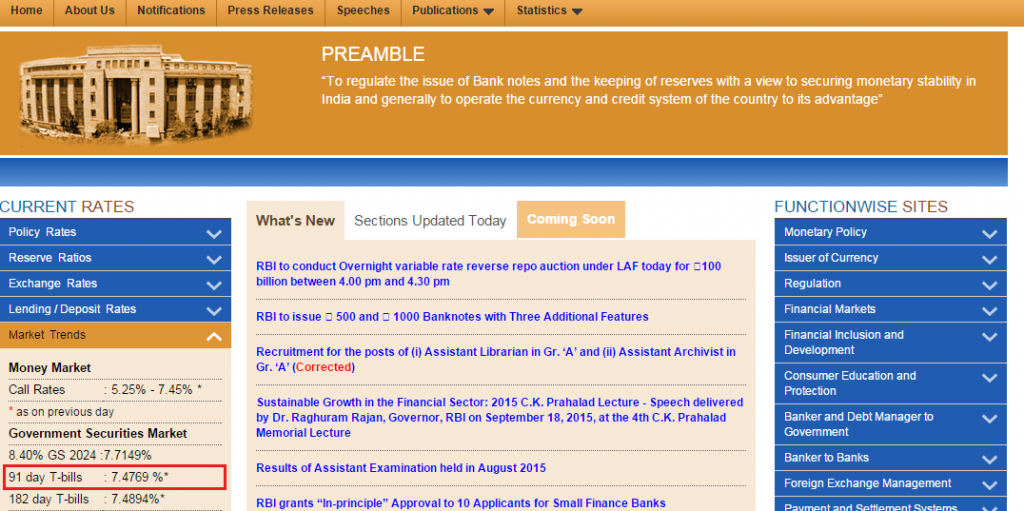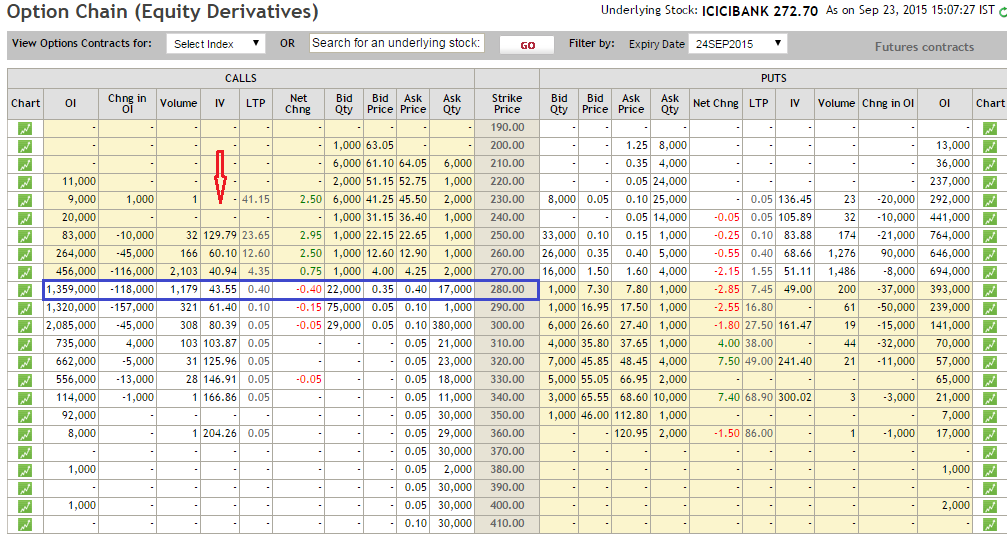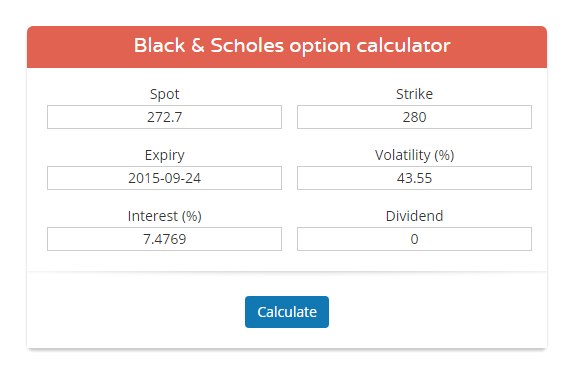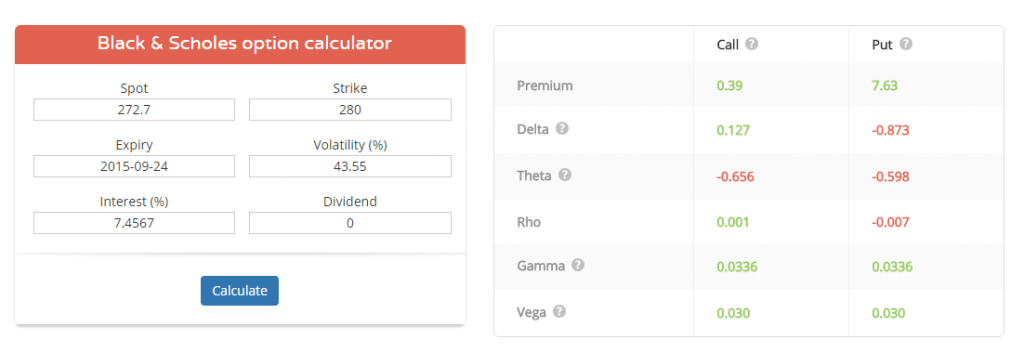# 21. Greek Calculator

## 21.1 – Background

So far in this module we have discussed all the important Option Greeks and their applications. It is now time to understand how to calculate these Greeks using the Black & Scholes (BS) Options pricing calculator. The BS options pricing calculator is based on the Black and Scholes options pricing model, which was first published by Fisher Black and Myron Scholes (hence the name Black & Scholes) in 1973, however Robert C Merton developed the model and brought in a full mathematical understanding to the pricing formula.

This particular pricing model is highly revered in the financial market, so much so that both Robert C Merton and Myron Scholes received the 1997 Noble Prize for Economic Sciences. The B&S options pricing model involves mathematical concepts such as partial differential equations, normal distribution, stochastic processes etc. The objective in this module is not to take you through the math in B&S model; in fact you could look at this video from Khan Academy for the same –

My objective is to take you through the practical application of the Black & Scholes options pricing formula.

## 21.2 – Overview of the model

Think of the BS calculator as a black box, which takes in a bunch of inputs and gives out a bunch of outputs. The inputs required are mostly market data of the options contract and the outputs are the Option Greeks.

The framework for the pricing model works like this:

1. We input the model with Spot price, Strike price, Interest rate, Implied volatility, Dividend, and Number of days to expiry
2. The pricing model churns out the required mathematical calculation and gives out a bunch of outputs
3. The output includes all the Option Greeks and the theoretical price of the call and put option for the strike selected

The illustration below gives the schema of a typical options calculator:On the input side:

Spot price – This is the spot price at which the underlying is trading. Note we can even replace the spot price with the futures price. We use the futures price when the option contract is based on futures as its underlying. Usually the commodity and in some cases the currency options are based on futures. For equity option contacts always use the spot price.

Interest Rate – This is risk free rate prevailing in the economy. Use the RBI 91 day Treasury bill rate for this purpose. You can get the rate from the RBI website, RBI has made it available on their landing page, as highlighted below.As of September 2015 the prevailing rate is 7.4769% per annum.

Dividend – This is the dividend per share expected in the stock, provided the stock goes ex dividend within the expiry period. For example, assume today is 11th September and you wish to calculate the Option Greeks for the ICICI Bank option contract. Assume ICICI Bank is going ex dividend on 18th Sept with a dividend of Rs.4. The expiry for the September series is 24th September 2015, hence the dividend would be Rs.4. in this case.

Number of days to expiry – This the number of calendar days left to expiry

Volatility – This is where you need to enter the option’s implied volatility. You can always look at the option chain provided by NSE to extract the implied volatility data. For example, here is the snap shot of ICICI Bank’s 280 CE, and as we can see, the IV for this contract is 43.55%.Let us use this information to calculate the option Greeks for ICICI 280 CE.

• Spot Price = 272.7
• Interest Rate = 7.4769%
• Dividend = 0
• Number of days to expiry = 1 (today is 23rd September, and expiry is on 24th September)
• Volatility = 43.55%

Once we have this information, we need to feed this into a standard Black & Scholes Options calculator. We do have this calculator on our website – https://zerodha.com/tools/black-scholes , you can use the same to calculate the Greeks.Once you enter the relevant data in the calculator and click on ‘calculate’, the calculator displays the Option Greeks –On the output side, notice the following –

• The premium of 280 CE and 280 PE is calculated. This is the theoretical option price as per the B&S options calculator. Ideally this should match with the current option price in the market
• Below the premium values, all the Options Greeks are listed.

I’m assuming that by now you are fairly familiar with what each of the Greeks convey, and the application of the same.

One last note on option calculators – the option calculator is mainly used to calculate the Option Greeks and the theoretical option price. Sometimes small difference arises owing to variations in input assumptions. Hence for this reason, it is good to have room for the inevitable modeling errors. However by and large, the option calculator is fairly accurate.

## 21.3 – Put Call Parity

While we are discussing the topic on Option pricing, it perhaps makes sense to discuss  ‘Put Call Parity’ (PCP). PCP is a simple mathematical equation which states –

Put Value + Spot Price = Present value of strike (invested to maturity) + Call Value.

The equation above holds true assuming –

1. Both the Put and Call are ATM options
2. The options are European
3. They both expire at the same time
4. The options are held till expiry

For people who are not familiar with the concept of Present value, I would suggest you read through this – http://zerodha.com/varsity/chapter/dcf-primer/ (section 14.3).

Assuming you are familiar with the concept of Present value, we can restate the above equation as –

P + S = Ke(-rt) + C

Where, Ke(-rt) represents the present value of strike, with K being the strike itself. In mathematical terms, strike K is getting discounted continuously at rate of ‘r’ over time‘t’

Also, do realize if you hold the present value of the strike and hold the same to maturity, you will get the value of strike itself, hence the above can be further restated as –

Put Option + Spot Price = Strike + Call options

• Trader A holds ATM Put option and 1 share of the underlying stock (left hand side of PCP equation)
• Trader B holds a Call option and cash amount equivalent to the strike (right hand side of PCP equation)

This being the case, as per the PCP the amount of money both traders make (assuming they hold till expiry) should be the same. Let us put some numbers to evaluate the equation –

Underlying = Infosys
Strike = 1200
Spot = 1200

Trader A holds = 1200 PE + 1 share of Infy at 1200
Trader B holds = 1200 CE + Cash equivalent to strike i.e 1200

Assume upon expiry Infosys expires at 1100, what do you think happens?

Trader A’s Put option becomes profitable and he makes Rs.100 however he loses 100 on the stock that he holds, hence his net pay off is 100 + 1100 = 1200.

Trader B’s Call option becomes worthless, hence the option’s value goes to 0, however he has cash equivalent to 1200, hence his account value is 0 + 1200 = 1200.

Let’s take another example, assume Infy hits 1350 upon expiry, lets see what happens to the accounts of both the trader’s.

Trader A = Put goes to zero, stock goes to 1350/-
Trader B = Call value goes to 150 + 1200 in cash = 1350/-

So clearly, irrespective of where the stock expires, the equations hold true, meaning both trader A and trader B end up making the same amount of money.

All good, but how would you use the PCP to develop a trading strategy? Well, for that you will have to wait for the next module which is dedicated to “Option Strategies” J. Before we start the next module on Option Strategies, we have 2 more chapters to go in this module.

### Key takeaways from this chapter

1. The options calculator is based on the Black & Scholes model
2. The Black & Scholes model is used to estimate the option’s theoretical price along with the option’s Greek
3. The interest rate in the B&S calculator refers to the risk free rate as available on the RBI site
4. The implied volatility can be fetched from the option chain from the NSE website
5. The put call parity states that the payoff from a put option plus the spot equals the payoff from call option plus the strike.

1.Shanmukha says:

When can we expect Option Strategies Module..?

•Karthik Rangappa says:

Very soon, another 2 chapters in the current module and we move to Options Strategies.

2.NARSIMHA says:

sir,what happens to overnight futures position in case of stock split like adani ent,idfc today

3.Ajay says:

I notice that the delta of 280 PE is -0.873. But, only deep ITM have such high deltas, right?

•Karthik Rangappa says:

Where did you get this value from Ajay?. Delta should be less than -0.5.

•Ajay says:

Think i got my answer. By the way, eagerly waiting for the next chapter. 🙂

•Karthik Rangappa says:

Next chapter (22) will be uploaded next week Ajay.

4.narsimha says:

sir,why should we use calender days in calculating theoratical option value,when trading days excluded whats use,enlighten me

•Karthik Rangappa says:

Well, the number of days is treated on something called as the ‘day count convention’…NSE adopts a day count convention called as ‘actual by actual’ according to which you need to take 365 days.

5.S gopalkrishna says:

Hi In the black scholes option calculator the input for IV is one but the theoretical prices for both put and call are computed based on that one IV INPUT Whereas in reality the IV puts and call for the same strike differ, so using which IV can be better? Can we use the VIX % instead?

•Karthik Rangappa says:

Vix is a good approximation for Nifty. For stock specific IV check the stock’s option chain for IV.